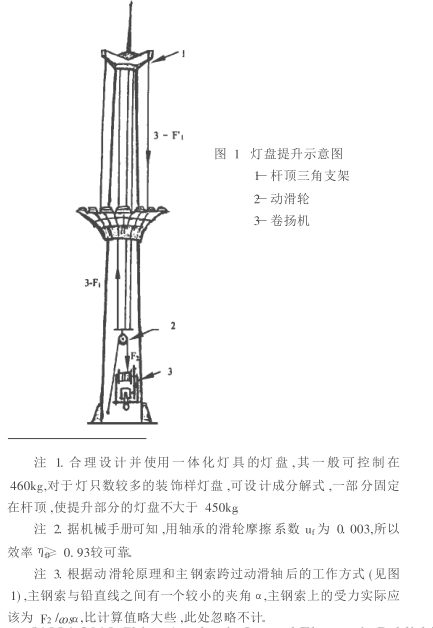18944481288# 30m高杆灯灯盘提升系统动力传递的设计计算

1、灯盘的提升方法2、动力传递的设计计算

2. 1三根分钢索上作用力分别为 F1 :F1 = ( 460÷ 3) /( 0. 93× 0. 93) × 9. 8= 1 737N

2. 2跨过提升铁板的主钢索盘绕在卷扬机组轮轴的一段受到的作用力 F2 :F2 =3F 12/Z0 =3× 17372/0. 93= 2802 N〈注 3〉

2.3主钢索选用直径 为 7. 7mm, 横 截 面积为22. 37mm2, 抗 拉 强 度 155kg /mm2 , (即1 519N /mm2) ,能承受最大拉力 33 908N。 现提升拉力F 2 =2 802N.使用中的安全系数 β= 33908/2802= 12. 1> 8。 完全符合国家有关部门规定。

2.4卷扬机的主体结构和关键尺寸。 卷扬机组的轮轴直径0 = 110mm,可排绕主钢索的长度 L= 215mm。 30m高杆灯 ,灯盘上升或下降的最大行程为 28m。 主钢索在轮轴上盘绕部分的总长度 ,即为主钢索跨过动滑轮移动的长度 S= 56m。 据计算: 56m主钢索在轮轴上需排绕 6层。 排绕第 1层时 ,轮轴加钢索的力学计算直径 O′0 = 110+ 7. 7= 117. 7mm;排绕第 6层时 ,轮轴加钢索后的力学计算直径 O″ 0 为190mm。 设这两种情况时 ,轮轴需付出的最小、最大扭矩分别为 M 小 和 M 大 :

M 小= F2 · ′ 0 /2= 2 802× 0. 118/2= 165. 3Nm( ′ 0 = 117. 7mm 0. 118m)M 大 = F 2 · ″ 0 /2= 2 802× 0. 19/2= 266. 2Nm

2.5卷扬机的轮轴与大齿轮同轴心组合。 大齿轮的分度圆直径  1 = 280mm,齿数为 70;与大齿轮衔接的小齿轮 (组装在减速机的输出轴心上 )分度圆直径2 = 192mm,齿数为 48。 两个传递齿轮的模数皆为4。 齿轮间的传递效率 η 2 = 0. 95。

2.6　 因大齿轮与轮轴同心组合 ,大齿轮分度圆周上必须付出的力矩等于 M 小或 M大 。设大齿轮分度圆周上承担的最大作用力为 F 3 :F3 = M 大 /12= 266. 2 / 0. 282= 1 901. 4N;

2.7小齿轮与大齿轮衔接 ,小齿轮必须付出的作用力为 F 4 ,付出的力矩 M:F4= F3/η2 = 1 901. 4/0. 95= 2 001. 5N;M= F 4 ·  2 /2= 2 001. 5× 0. 192 /2= 192. 1Nm

2.8若选用 W PA80型减速机 ,其输入转速为 800r /min,输出扭矩为 211Nm,减速比为 62∶ 1,因 211Nm> M( 192. 1Nm) ,符合需求。